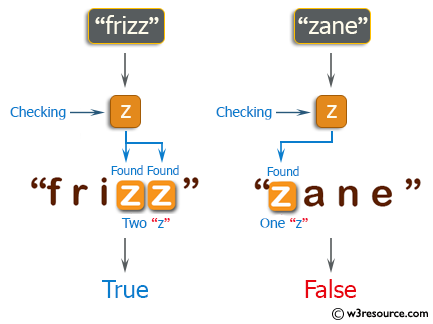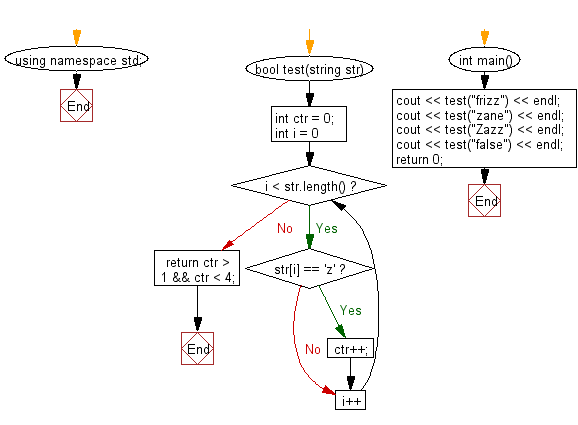﻿ C++ : Check if a string contains a character between 2 and 4# C++ Exercises: Check if a given string contains between 2 and 4 'z' character

## C++ Basic Algorithm: Exercise-22 with Solution

Write a C++ program to check if a given string contains between 2 and 4 'z' character.

Sample Solution:

C++ Code :

``````#include <iostream>

using namespace std;

bool test(string str)
{
int ctr = 0;

for (int i = 0; i < str.length(); i++)
{
if (str[i] == 'z')
{
ctr++;
}
}

return ctr > 1 && ctr < 4;
}

int main()
{
cout << test("frizz") << endl;
cout << test("zane") << endl;
cout << test("Zazz") << endl;
cout << test("false") << endl;
return 0;
}
``````

Sample Output:

```1
0
1
0
```

Pictorial Presentation:Flowchart:C++ Code Editor: